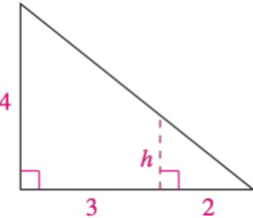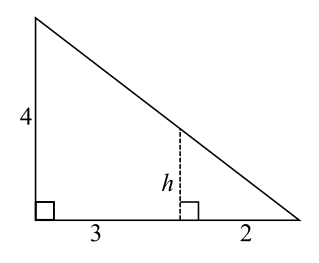Chapter 8.1, Problem 40E### Calculus: An Applied Approach (Min...

10th Edition
Ron Larson
ISBN: 9781305860919

#### Solutions

Chapter
Section### Calculus: An Applied Approach (Min...

10th Edition
Ron Larson
ISBN: 9781305860919
Textbook Problem
1 views

# Analyzing Triangles In Exercises 33-40, solve the triangle for the indicated side and/or angle.To determine

To calculate: The value of side h in a triangle provided below.Explanation

Given Information:

The provided triangle is shown below,

Formula used:

When two triangles are similar or have equal angles then the ratio the two sides are equal and corresponding angles opposite to these sides are also equal.

Calculation:

Consider the triangle provided below.

From above diagram, the larger triangle is similar to the smaller triangle ΔABCΔEDC.

So, the unknown side h can be calculated by using property of similar triangles

### Still sussing out bartleby?

Check out a sample textbook solution.

See a sample solution

#### The Solution to Your Study Problems

Bartleby provides explanations to thousands of textbook problems written by our experts, many with advanced degrees!

Get Started

#### Describe the set of points (x,y) such that x2+y2=0.

Finite Mathematics and Applied Calculus (MindTap Course List)

#### In Exercises 73-80, find the indicated limits, if they exist. 78. limx4x43x2+12x4+x3+x2+x+1

Applied Calculus for the Managerial, Life, and Social Sciences: A Brief Approach

#### In problems 23-58, perform the indicated operations and simplify. 45.

Mathematical Applications for the Management, Life, and Social Sciences

#### Prove the idempotent law for conjunction, ppp.

Finite Mathematics for the Managerial, Life, and Social Sciences

#### True or False: converges conditionally.

Study Guide for Stewart's Multivariable Calculus, 8th

#### True or False: converges.

Study Guide for Stewart's Single Variable Calculus: Early Transcendentals, 8th